# Answer the following question that exploits the understanding of Logic and Gates. Your simplified answer should...

Answer the following question that exploits the understanding of Logic and Gates. Your simplified answer should use 3 gates.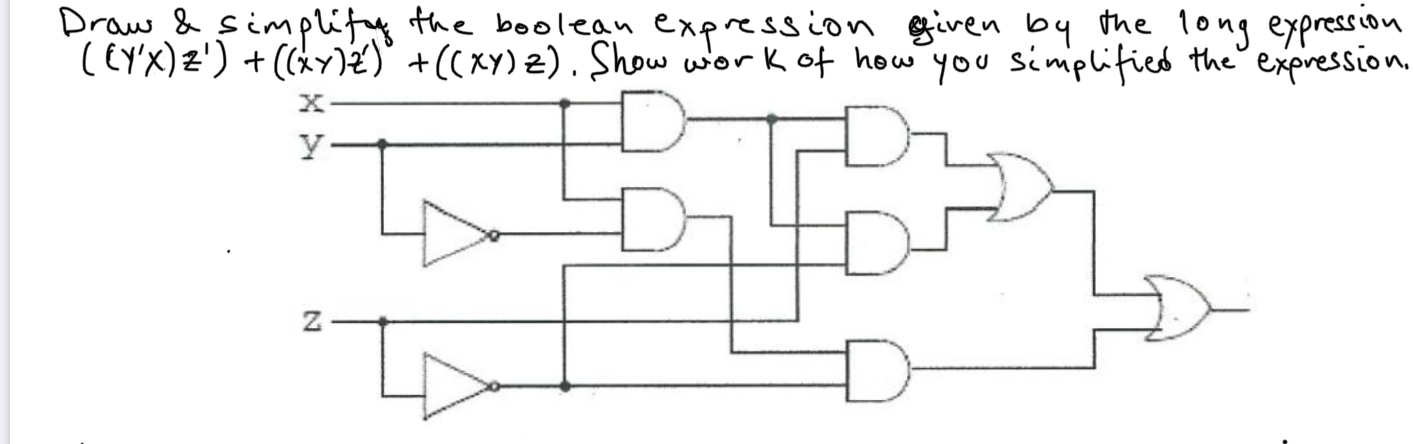Draw & simplify the boolean expression given by the long expression (LY'X)Z') + ((*y) )° +((XY) 2). Show work of how you simplified the expression,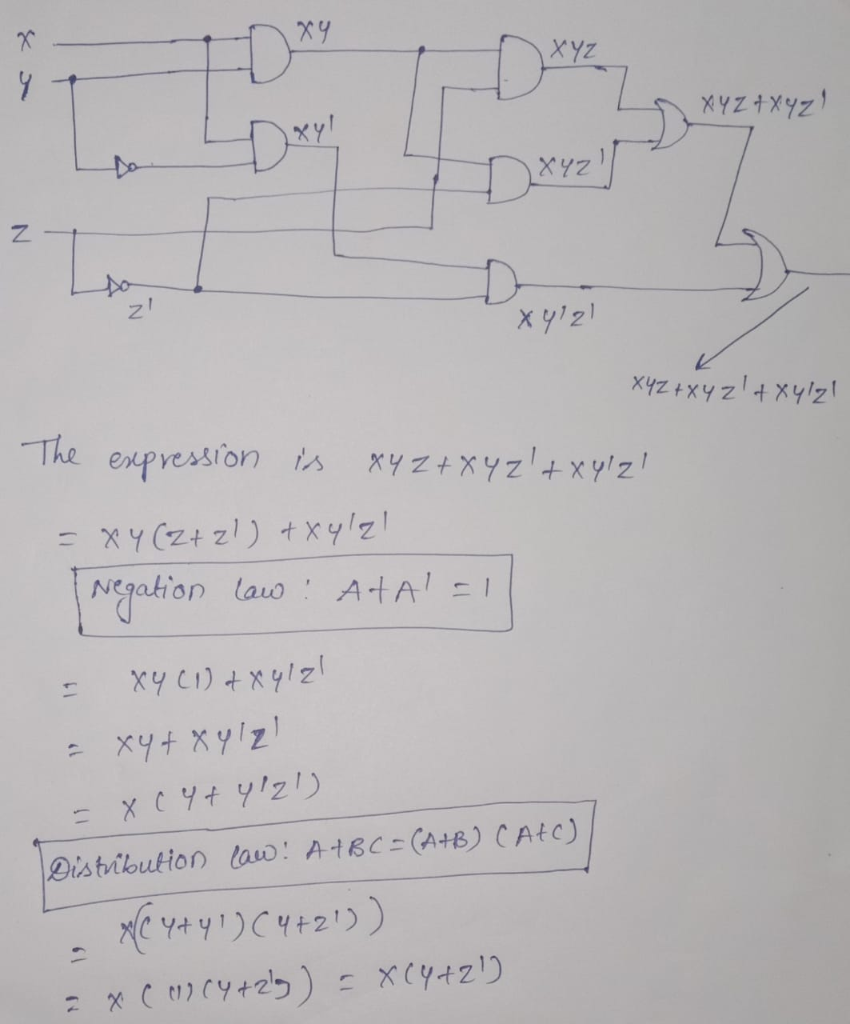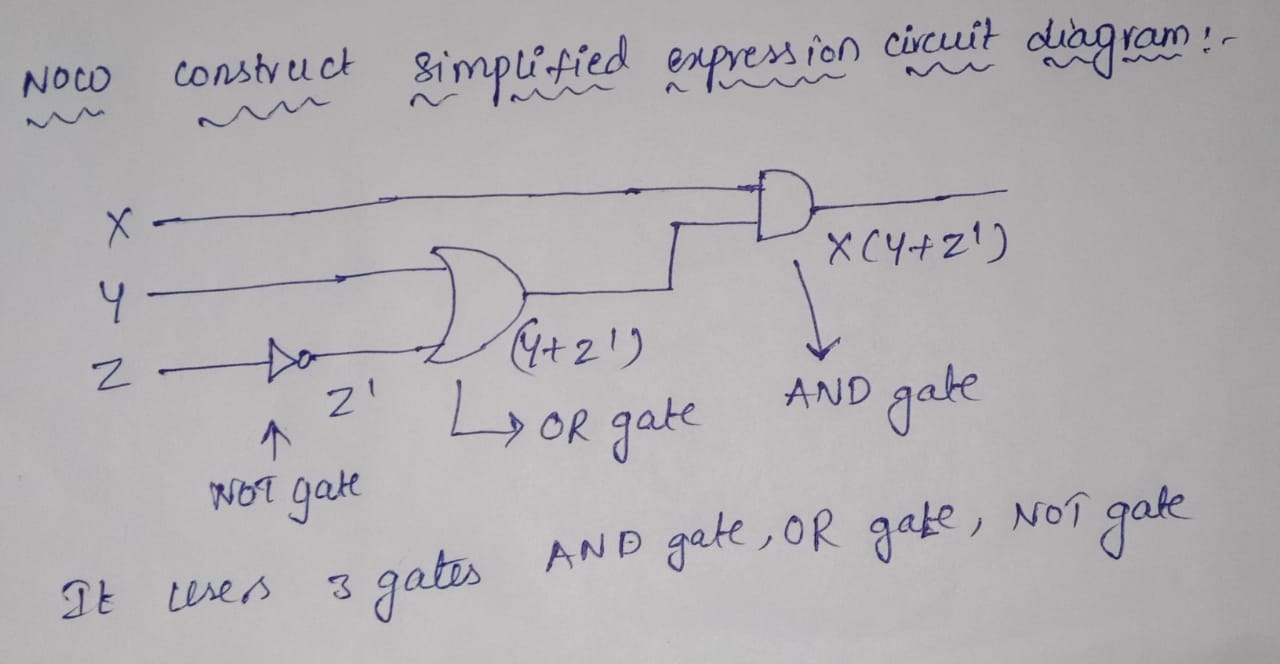#### Earn Coin

Coins can be redeemed for fabulous gifts.

Similar Homework Help Questions
• ### Using the Boolean logic expression below, draw circuit diagram with logic gates that will implement your...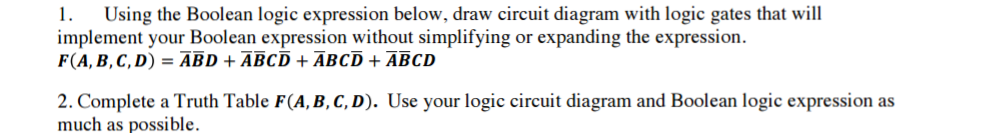Using the Boolean logic expression below, draw circuit diagram with logic gates that will implement your Boolean expression without simplifying or expanding the expression. F(A, B, C, D) = ABD + ABCD + ABCD + ABCD Complete a Truth Table F(A, B, C, D). Use your logic circuit diagram and Boolean logic expression as much as possible.

• ### 1. What logic gates are known as universal gates? (1 point) a) nand, nor b) and,...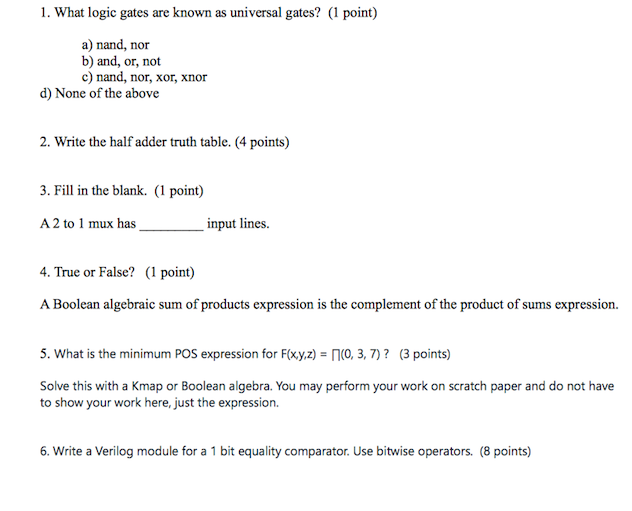1. What logic gates are known as universal gates? (1 point) a) nand, nor b) and, or, not c) nand, nor, xor, xnor d) None of the above 2. Write the half adder truth table. (4 points) 3. Fill in the blank. (1 point) A2 to 1 mux has input lines. 4. True or False? (1 point) A Boolean algebraic sum of products expression is the complement of the product of sums expression. 5. What is the minimum POS expression...

• ### 2.18 For the Boolean function F = xyz + x'y'z + w xy + wx'y +...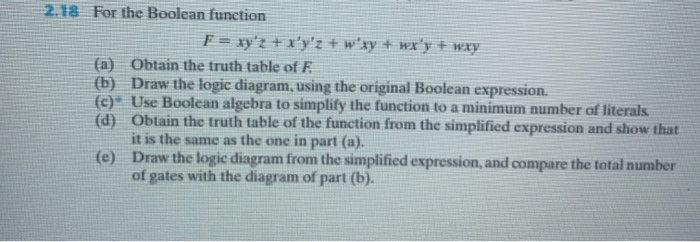2.18 For the Boolean function F = xyz + x'y'z + w xy + wx'y + wxy (a) Obtain the truth table of E (b) Draw the logic diagram, using the original Boolean expression. (c) Use Boolean algebra to simplify the function to a minimum number of literals (d) Obtain the truth table of the function from the simplified expression and show that it is the same as the one in part (a). (e) Draw the logic diagram from the...

• ### 6. Convert .3710 to a binary fraction of 10 binary digits. 7. Use two's compliment arithmetic to perform the following 8 bit binary operations. a. 0010 1110 + 0001 1011 b. 0101 1101 – 0011 1010 c....

6. Convert .3710 to a binary fraction of 10 binary digits. 7. Use two's compliment arithmetic to perform the following 8 bit binary operations. a. 0010 1110 + 0001 1011 b. 0101 1101 – 0011 1010 c. 1011 1000 – 1000 1011 d. 1000 1100 – 1111 0111 8. Convert 150.8476562510 to IEEE Floating Point Standard. 9. Simplify the following Boolean expressions. a. xy + xy + xz b. (w + x)(x + y)(w + x + y + z)...

• ### Question 3: For the logic circuit given below: A (3 pts) Write Boolean expression for output...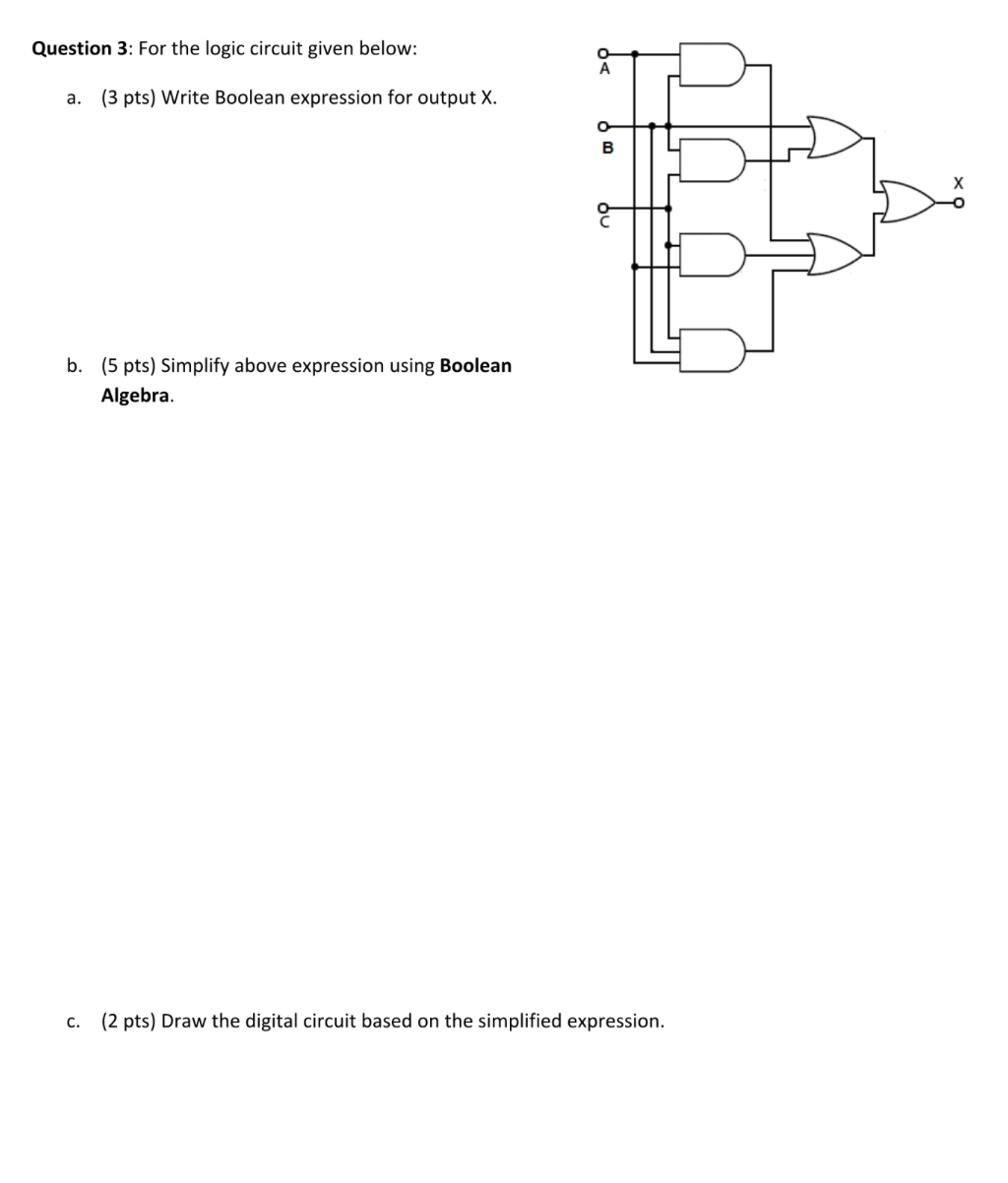Question 3: For the logic circuit given below: A (3 pts) Write Boolean expression for output X. a. B b. (5 pts) Simplify above expression using Boolean Algebra (2 pts) Draw the digital circuit based on the simplified expression. C.

• ### Given the following boolean expression: F = ABC + ABC + ABC 1. Simplify the expression...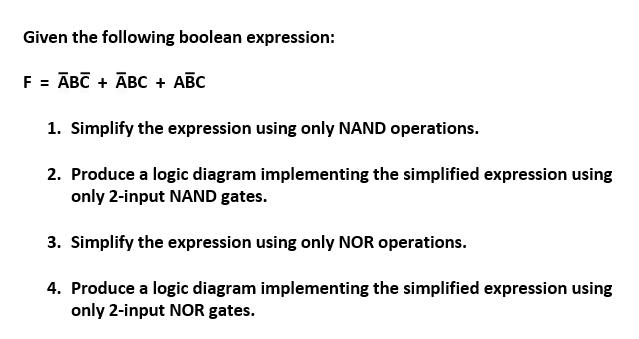Given the following boolean expression: F = ABC + ABC + ABC 1. Simplify the expression using only NAND operations. 2. Produce a logic diagram implementing the simplified expression using only 2-input NAND gates. 3. Simplify the expression using only NOR operations. 4. Produce a logic diagram implementing the simplified expression using only 2-input NOR gates.

• ### Implement this Boolean Expression on a breadboard using NOR gates Part A: Z =XY+X 'Y' Implement...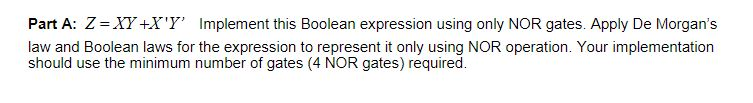Implement this Boolean Expression on a breadboard using NOR gates Part A: Z =XY+X 'Y' Implement this Boolean expression using only NOR gates. Apply De Morgan's law and Boolean laws for the expression to represent it only using NOR operation. Your implementation should use the minimum number of gates (4 NOR gates) required

• ### 1. Simplify the following Boolean expression: (solution should be one term) XY+XY 2. Simplify the following...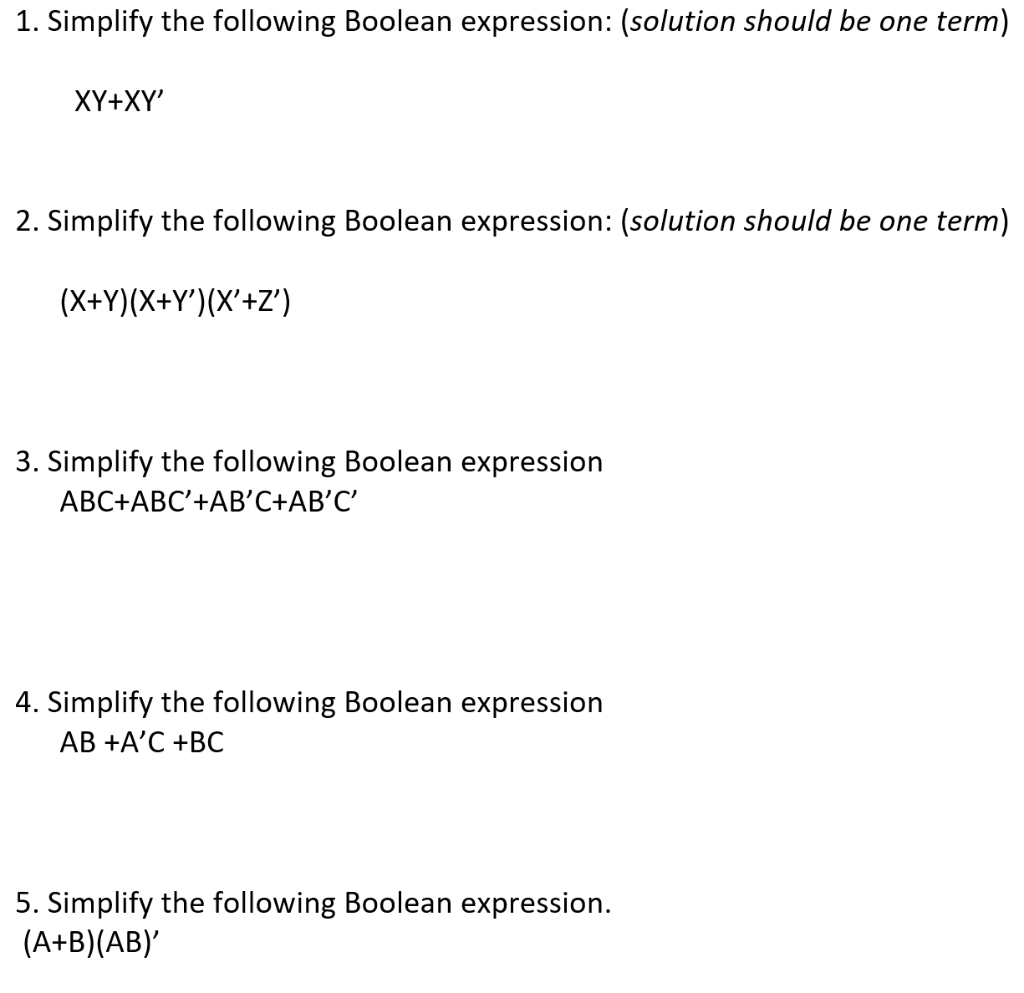1. Simplify the following Boolean expression: (solution should be one term) XY+XY 2. Simplify the following Boolean expression: (solution should be one term) (X+Y)(X+Y)(X'+Z”) 3. Simplify the following Boolean expression ABC+ABC'+AB'C+AB'C' 4. Simplify the following Boolean expression AB +A'C +BC 5. Simplify the following Boolean expression. (A+B)(AB)

• ### Given the function F(x,y,z) = xyztx,y2+xyz (a) List the truth table for F (b) Draw the...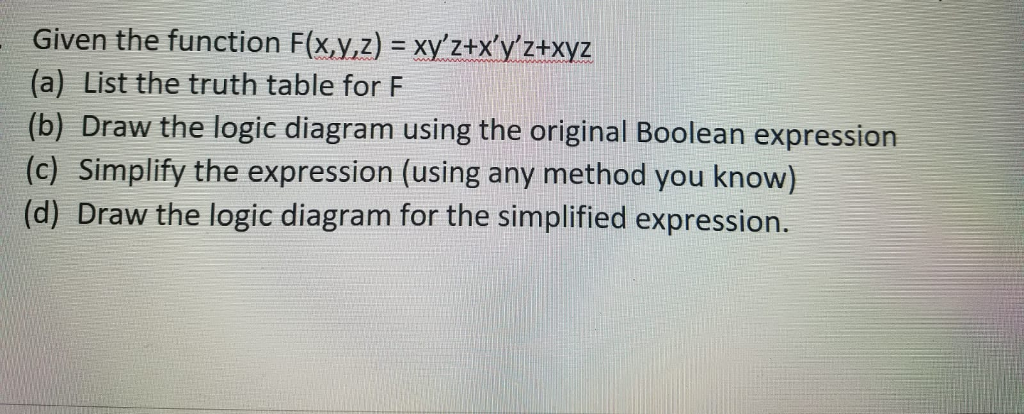Given the function F(x,y,z) = xyztx,y2+xyz (a) List the truth table for F (b) Draw the logic diagram using the original Boolean expression (c) Simplify the expression (using any method you know) (d) Draw the logic diagram for the simplified expression.

• ### 8).- The logic circuit shown is used to turn on a warning buzzer at X based...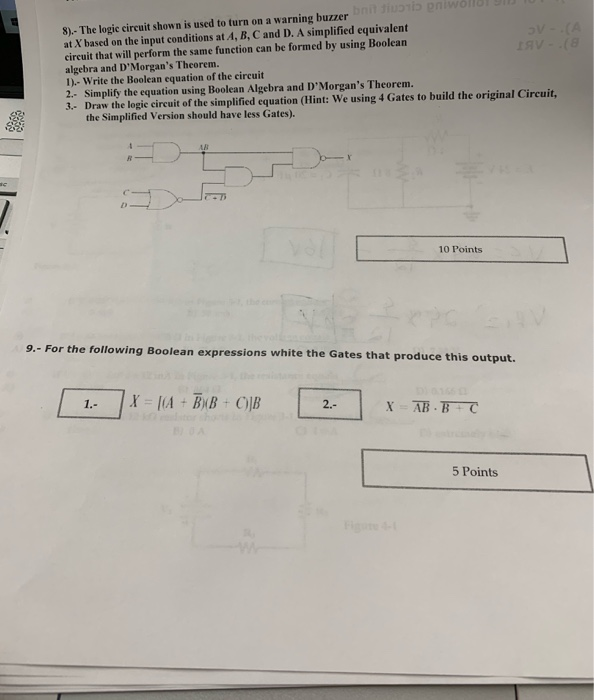8).- The logic circuit shown is used to turn on a warning buzzer at X based on the input conditions at A, B, C and D. A simplified equivalent circuit that will perform the same function can be formed by using Boolean algebra and D'Morgan's Theorem. 1).- Write the Boolean equation of the circuit 2. Simplify the equation using Boolean Algebra and D'Morgan's Theorem. 3. Draw the logie circuit of the simplified equation (Hint: We using 4 Gates to build...